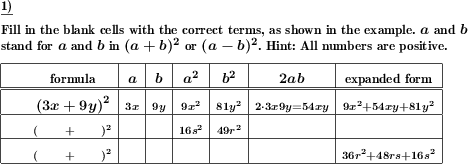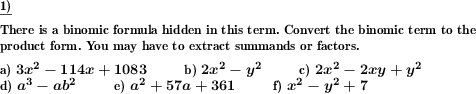Custom math worksheets at your fingertips# Details for problem "Examine the elements of binomic formulas"

Quickname: 7596

Elementary School, Primary School, Junior High School, Middle School, High School.

## Summary

Elements of a binomic formula and their expanded form are grouped in a table. Fill the empty spaces.

## Example## Description

In a tabular form, structural elements of binomic formulas in parentheses and expanded form like

• binomic formular in parentheses form
• a
• b
• 2ab
• a^2
• b^2
• expanded form

are shown as captions for a table. The task is, for a given binomic formula, to identify these structural elements and fill in the table's columns accordingly.

In every row of the table, depending on the problem statement, some elements will be given, some will be left blank to be filled in.

For the problem generation, it is selectable if a and b will be simple literal numbers or variables or multiples of variables. The first problem may serve as an example and will then presented in the form of a fully populated row.

The number of problems is selectable, and the types of formulas to be used.

## Free worksheets and solution sheets with answers for download

Download free printable worksheets for this math problem here. The worksheet contains the problems only, the solution sheet includes the answers. Just click on the respective link.

•Worksheet 1Solution sheet with answers
•Worksheet 2Solution sheet with answers
•Worksheet 3Solution sheet with answers

If you can not see the solution sheets for download, they may be filtered out by an ad blocker that you may have installed. If this is the case, please allow ads for this page and reload the page. The solution sheets will then reappear.

• Do these sample worksheets do not really fit?
• Do you need more math worksheets, with a different level of difficulty?
• Would you like to combine different problems on a worksheet and adjust them to your needs?
• As a teacher, you can put together your own worksheets using the automatically generated math problems provided.
With a free initial credit, you can start creating your own math worksheets in a few minutes.

You can try it for free! Register here, to create custom worksheets now!

## Customization options for this problem

Parameter
Possible values
Number of problems
1, 2, 3, 4, 5, 6, 7, 8, 9, 10
Type of formula
(a+b)^2 & (a-b)^2, (a+b)(a-b)
Columns provided
parentheses form, expanded, a,b, a^2, b^2, mix of a,a^2,b,b^2, random
Summands
simple var/number, product of var/number
Sample problem
Yes, No

## Similar problems

Remark
Description
Name and direct link
Expansion of simple binomic formulas
Apply binomic formula expansion rules to simplify term.
Expansion of binomic formulas with complex terms
Apply binomic formula expansion rules to simplify term. Advanced version mit multiples of variables as summands.

## dw-Math worksheet templates that do contain this problem

Name
Title
Description
Binomic formulas: Advanced applications
Use the binomic formulas to simplify and expand terms.

## Other types of problems that appear on worksheets with this problem:

Relevance
Name
Description
Quickname
Example
****
Expand and simplify binomic formula, advanced
Apply binomic formula expansion rules to simplify term. Advanced version mit multiples of variables as summands.****
Extract binomic formula from term
A binomic formula is hidden in a term. Factor out the binomic formular or extract the summand.****
Simplify term with binomic formula, combine like terms
Simplify a term which contains a binomic formulaYou may pass on the worksheets offered for download on this page to others, but not modify or sell them.